# polypoly package overview

## Background

The poly() function in the stats package creates a matrix of (orthogonal) polynomials over a set of values. The code below shows some examples of these matrices.

# orthogonal polynomials
m <- poly(1:6, degree = 3, simple = TRUE)
m
#>               1          2          3
#> [1,] -0.5976143  0.5455447 -0.3726780
#> [2,] -0.3585686 -0.1091089  0.5217492
#> [3,] -0.1195229 -0.4364358  0.2981424
#> [4,]  0.1195229 -0.4364358 -0.2981424
#> [5,]  0.3585686 -0.1091089 -0.5217492
#> [6,]  0.5976143  0.5455447  0.3726780

# the terms are uncorrelated. that's why they are "orthogonal".
zapsmall(cor(m))
#>   1 2 3
#> 1 1 0 0
#> 2 0 1 0
#> 3 0 0 1

# raw polynomials
m <- poly(1:6, degree = 3, simple = TRUE, raw = TRUE)
m
#>      1  2   3
#> [1,] 1  1   1
#> [2,] 2  4   8
#> [3,] 3  9  27
#> [4,] 4 16  64
#> [5,] 5 25 125
#> [6,] 6 36 216

# raw polynomials are highly correlated.
round(cor(m), 2)
#>      1    2    3
#> 1 1.00 0.98 0.94
#> 2 0.98 1.00 0.99
#> 3 0.94 0.99 1.00

This package provides some helpful functions for working with these matrices.

## Examples

### Tidying

This package provides a tidying function poly_melt().

library(polypoly)
xs <- 1:40
poly_mat <- poly(xs, degree = 5)
poly_melt(poly_mat)
#> # A tibble: 200 × 3
#>    observation degree  value
#>          <int> <chr>   <dbl>
#>  1           1 1      -0.267
#>  2           2 1      -0.253
#>  3           3 1      -0.240
#>  4           4 1      -0.226
#>  5           5 1      -0.212
#>  6           6 1      -0.199
#>  7           7 1      -0.185
#>  8           8 1      -0.171
#>  9           9 1      -0.158
#> 10          10 1      -0.144
#> # … with 190 more rows

The returned dataframe has one row per cell of the original matrix. Essentialy, the columns of the matrix are stacked on top of each other to create a long dataframe. The observation and degree columns record each values’ original row number and column name, respectively.

### Plotting

Plot a matrix with poly_plot().

poly_plot(poly_mat)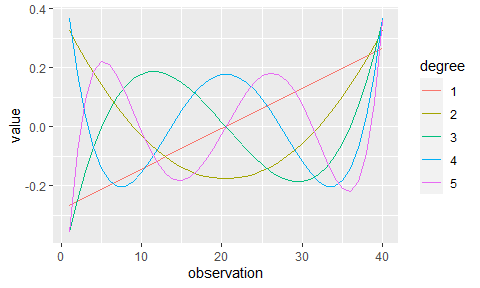We can also plot raw polynomials, but that display is less useful because the x-axis corresponds to the row number of polynomial matrix.

poly_raw_mat <- poly(-10:10, degree = 3, raw = TRUE)
poly_plot(poly_raw_mat)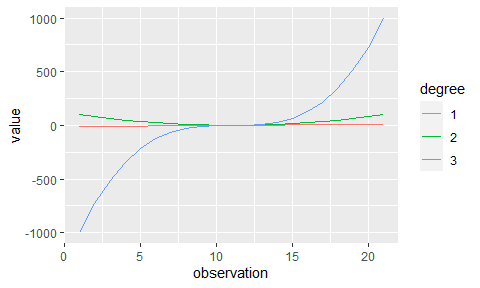We can make the units clearer by using by_observation = FALSE so that the x-axis corresponds to the first column of the polynomial matrix.

poly_plot(poly_raw_mat, by_observation = FALSE)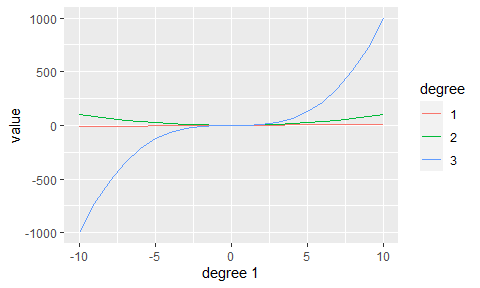poly_plot() returns a plain ggplot2 plot, so we can further customize the output. For example, we can use ggplot2 to compute the sum of the individual polynomials and re-theme the plot.

library(ggplot2)
poly_plot(poly_mat) +
stat_summary(
aes(color = "sum"),
fun = "sum",
geom = "line",
size = 1
) +
theme_minimal()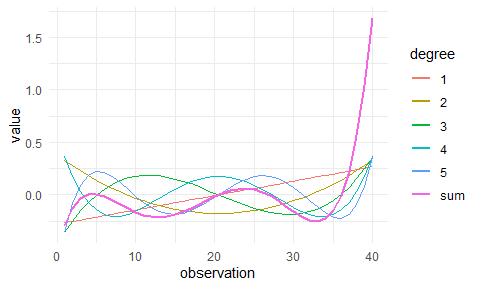For total customization, poly_plot_data() will return the dataframe that would have been plotted by poly_plot().

poly_plot_data(poly_mat, by_observation = FALSE)
#> # A tibble: 200 × 4
#>    observation degree   value degree 1
#>          <int> <fct>    <dbl>      <dbl>
#>  1           1 1      -0.267      -0.267
#>  2           1 2       0.328      -0.267
#>  3           1 3      -0.360      -0.267
#>  4           1 4       0.369      -0.267
#>  5           1 5      -0.360      -0.267
#>  6           2 5      -0.0831     -0.253
#>  7           2 1      -0.253      -0.253
#>  8           2 2       0.278      -0.253
#>  9           2 3      -0.249      -0.253
#> 10           2 4       0.180      -0.253
#> # … with 190 more rows

### Rescaling a matrix

The ranges of the terms created by poly() are sensitive to repeated values.

# For each column in a matrix, return difference between max and min values
col_range <- function(matrix) {
apply(matrix, 2, function(xs) max(xs) - min(xs))
}

p1 <- poly(0:9, degree = 2)
p2 <- poly(rep(0:9, 18), degree = 2)

col_range(p1)
#>         1         2
#> 0.9908674 0.8703883
col_range(p2)
#>         1         2
#> 0.2335497 0.2051525

Thus, two models fit with y ~ poly(x, 3) will not have comparable coefficients when the number of rows changes, even if the unique values of x did not change!

poly_rescale() adjusts the values in the polynomial matrix so that the linear component has a specified range. The other terms are scaled by the same factor.

col_range(poly_rescale(p1, scale_width = 1))
#>         1         2
#> 1.0000000 0.8784105
col_range(poly_rescale(p2, scale_width = 1))
#>         1         2
#> 1.0000000 0.8784105

poly_plot(poly_rescale(p2, scale_width = 1), by_observation = FALSE)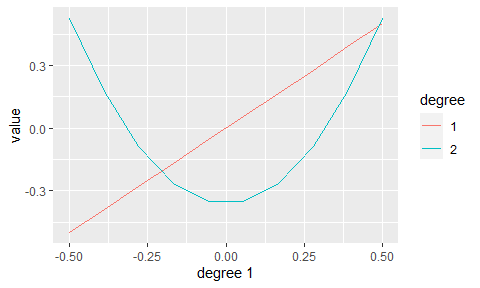### Adding columns to a dataframe

poly_add_columns() adds orthogonal polynomial transformations of a predictor variable to a dataframe.

Here’s how we could add polynomials to the sleepstudy dataset.

df <- tibble::as_tibble(lme4::sleepstudy)
print(df)
#> # A tibble: 180 × 3
#>    Reaction  Days Subject
#>       <dbl> <dbl> <fct>
#>  1     250.     0 308
#>  2     259.     1 308
#>  3     251.     2 308
#>  4     321.     3 308
#>  5     357.     4 308
#>  6     415.     5 308
#>  7     382.     6 308
#>  8     290.     7 308
#>  9     431.     8 308
#> 10     466.     9 308
#> # … with 170 more rows

#> # A tibble: 180 × 6
#>    Reaction  Days Subject   Days1   Days2  Days3
#>       <dbl> <dbl> <fct>     <dbl>   <dbl>  <dbl>
#>  1     250.     0 308     -0.495   0.522  -0.453
#>  2     259.     1 308     -0.385   0.174   0.151
#>  3     251.     2 308     -0.275  -0.0870  0.378
#>  4     321.     3 308     -0.165  -0.261   0.335
#>  5     357.     4 308     -0.0550 -0.348   0.130
#>  6     415.     5 308      0.0550 -0.348  -0.130
#>  7     382.     6 308      0.165  -0.261  -0.335
#>  8     290.     7 308      0.275  -0.0870 -0.378
#>  9     431.     8 308      0.385   0.174  -0.151
#> 10     466.     9 308      0.495   0.522   0.453
#> # … with 170 more rows

We can optionally customize the column names and rescale the polynomial terms.

poly_add_columns(df, Days, degree = 3, prefix = "poly_", scale_width = 1)
#> # A tibble: 180 × 6
#>    Reaction  Days Subject  poly_1  poly_2 poly_3
#>       <dbl> <dbl> <fct>     <dbl>   <dbl>  <dbl>
#>  1     250.     0 308     -0.5     0.527  -0.458
#>  2     259.     1 308     -0.389   0.176   0.153
#>  3     251.     2 308     -0.278  -0.0878  0.381
#>  4     321.     3 308     -0.167  -0.264   0.338
#>  5     357.     4 308     -0.0556 -0.351   0.131
#>  6     415.     5 308      0.0556 -0.351  -0.131
#>  7     382.     6 308      0.167  -0.264  -0.338
#>  8     290.     7 308      0.278  -0.0878 -0.381
#>  9     431.     8 308      0.389   0.176  -0.153
#> 10     466.     9 308      0.5     0.527   0.458
#> # … with 170 more rows

We can confirm that the added columns are orthogonal.

df <- poly_add_columns(df, Days, degree = 3, scale_width = 1)
zapsmall(cor(df[c("Days1", "Days2", "Days3")]))
#>       Days1 Days2 Days3
#> Days1     1     0     0
#> Days2     0     1     0
#> Days3     0     0     1

### Experimental

#### Splines

This package also (accidentally) works on splines. Splines are not officially supported, but they could be an avenue for future development.

poly_plot(splines::bs(1:100, 10, intercept = TRUE))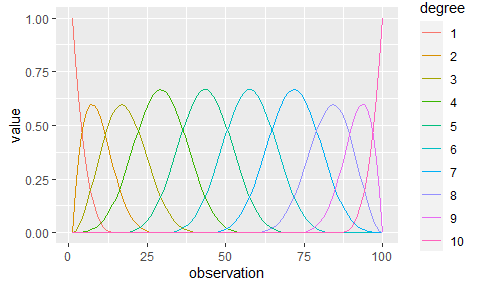poly_plot(splines::ns(1:100, 10, intercept = FALSE))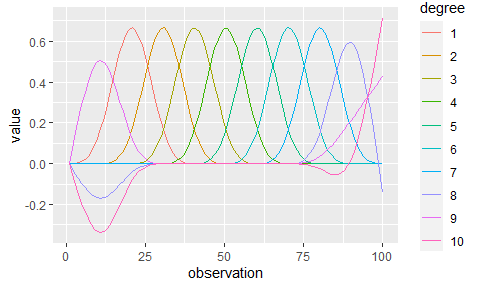## Longer example: Visualizing growth curve contributions

This section illustrates a use case that may or may not be included in the package someday: Visualizing the weighting of polynomial terms from a linear model. For now, here’s how to do that task with this package.

Suppose we want to model some change over time using a cubic polynomial. For example, the growth of trees.

library(lme4)
df <- tibble::as_tibble(Orange)
df$Tree <- as.character(df$Tree)
df
#> # A tibble: 35 × 3
#>    Tree    age circumference
#>    <chr> <dbl>         <dbl>
#>  1 1       118            30
#>  2 1       484            58
#>  3 1       664            87
#>  4 1      1004           115
#>  5 1      1231           120
#>  6 1      1372           142
#>  7 1      1582           145
#>  8 2       118            33
#>  9 2       484            69
#> 10 2       664           111
#> # … with 25 more rows

ggplot(df) +
aes(x = age, y = circumference, color = Tree) +
geom_line()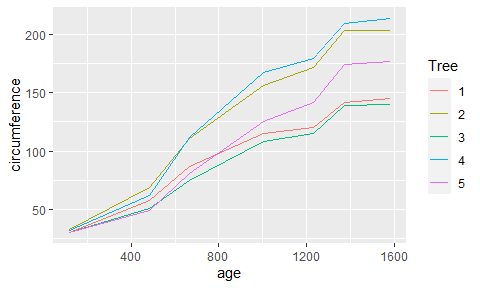We can bind the polynomial terms onto the data and fit a model.

df <- poly_add_columns(Orange, age, 3, scale_width = 1)

model <- lmer(
scale(circumference) ~ age1 + age2 + age3 + (age1 + age2 + age3 | Tree),
data = df
)
#> boundary (singular) fit: see help('isSingular')
summary(model)
#> Linear mixed model fit by REML ['lmerMod']
#> Formula: scale(circumference) ~ age1 + age2 + age3 + (age1 + age2 + age3 |
#>     Tree)
#>    Data: df
#>
#> REML criterion at convergence: -11.7
#>
#> Scaled residuals:
#>      Min       1Q   Median       3Q      Max
#> -1.54075 -0.42547  0.08553  0.49188  1.37391
#>
#> Random effects:
#>  Groups   Name        Variance Std.Dev. Corr
#>  Tree     (Intercept) 0.12584  0.35474
#>           age1        0.38473  0.62027   0.99
#>           age2        0.00997  0.09985  -0.63 -0.49
#>           age3        0.01070  0.10343  -0.89 -0.95  0.19
#>  Residual             0.01688  0.12991
#> Number of obs: 35, groups:  Tree, 5
#>
#> Fixed effects:
#>               Estimate Std. Error t value
#> (Intercept) -1.867e-14  1.602e-01   0.000
#> age1         2.719e+00  2.852e-01   9.533
#> age2        -1.520e-01  7.995e-02  -1.901
#> age3        -2.529e-01  8.085e-02  -3.128
#>
#> Correlation of Fixed Effects:
#>      (Intr) age1   age2
#> age1  0.950
#> age2 -0.348 -0.266
#> age3 -0.502 -0.529  0.062
#> optimizer (nloptwrap) convergence code: 0 (OK)
#> boundary (singular) fit: see help('isSingular')

How do we understand the contribution of each of these terms? We can recreate the model matrix by attaching the intercept term to a polynomial matrix.

poly_mat <- poly_rescale(poly(df$age, degree = 3), 1) # Keep only seven rows because there are 7 observations per tree poly_mat <- poly_mat[1:7, ] pred_mat <- cbind(constant = 1, poly_mat) pred_mat #> constant 1 2 3 #> [1,] 1 -0.54927791 0.5047675 -0.2964647 #> [2,] 1 -0.29927791 -0.1637435 0.4264971 #> [3,] 1 -0.17632709 -0.3312105 0.2957730 #> [4,] 1 0.05591335 -0.3573516 -0.2139411 #> [5,] 1 0.21096799 -0.1635520 -0.3699278 #> [6,] 1 0.30727947 0.0419906 -0.2489830 #> [7,] 1 0.45072209 0.4690995 0.4070466 Weight the predictors using the model fixed effects. weighted <- pred_mat %*% diag(fixef(model)) colnames(weighted) <- colnames(pred_mat) And do some tidying to plot the two sets of predictors. df_raw <- poly_melt(pred_mat) df_raw$predictors <- "raw"

df_weighted <- poly_melt(weighted)
df_weighted$predictors <- "weighted" df_both <- rbind(df_raw, df_weighted) # Only need the first 7 observations because that is one tree ggplot(df_both[df_both$observation <= 7, ]) +
aes(x = observation, y = value, color = degree) +
geom_line() +
facet_grid(. ~ predictors) +
labs(color = "term")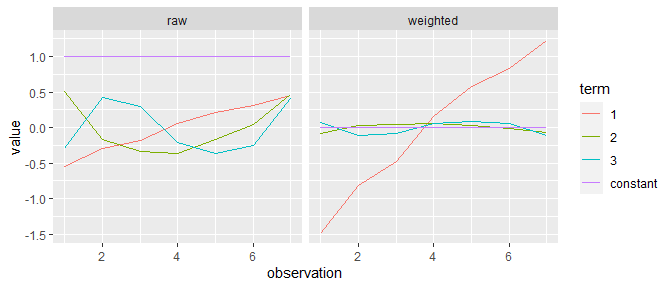The linear trend drives the growth curve. The quadratic and cubic terms make tiny contributions. We can see that the intercept term does nothing (because we used scale() in the model).

Hmmm… perhaps we need to find a better example dataset for this example.

## Resources

If you searched for help on poly(), see also: